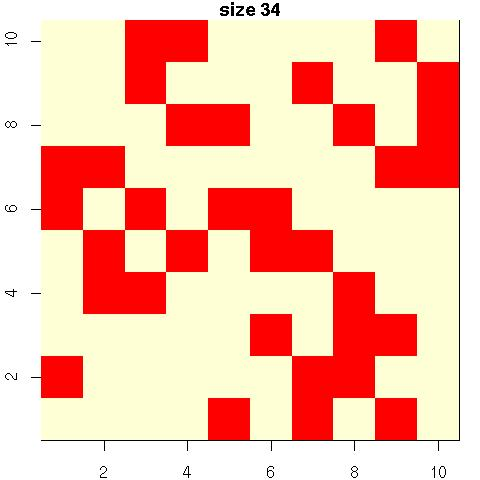Want to share your content on R-bloggers? click here if you have a blog, or here if you don't.

In the weekend supplement to Le Monde, the solution of the rectangle puzzle is given as 32 black squares. I am thus… puzzled!, since my R program there provides a 34 square solution.Am I missing a hidden rectangle in the above?! Given that the solution in Le Monde is not based on a precise mathematical argument (something to do with graph theory???), it may be that the authors of this puzzle got their reasoning wrong… (The case of the parallelogram is clearer, the argument being that an horizontal distance between two black squares can only occur once.) An open problem (?) is then to figure out a formula for the number of black squares on an nxn grid without any rectangle. (I wonder how this is linked with the friendly queen puzzle set by Gauß…)

Filed under: Kids, R Tagged: Le Monde, mathematical puzzle